# yline

## 语法

``yline(y)``
``yline(y,LineSpec)``
``yline(y,LineSpec,labels)``
``yline(___,Name,Value)``
``yline(ax,___)``
``yl = yline(___)``

## 说明

``yline(y)` 在当前坐标区的一个或多个 y 坐标处创建一条水平线。例如，`yline(2)` 在 `y=2` 处创建一条线。`

``yline(y,LineSpec)` 指定线型、线条颜色或同时指定两者。例如，`xline([12 20 33],'--b')` 创建三条蓝色虚线。`

``yline(y,LineSpec,labels)` 为线条添加标签。`

``yline(___,Name,Value)` 使用一个或多个名称-值对组参数指定常量线属性。有关属性列表，请参阅 ConstantLine 属性。请在所有其他输入参数之后指定名称-值对组。`

``yline(ax,___)` 在指定的坐标区（而不是当前坐标区）中创建线条。要确定当前坐标区，请使用 `gca`。 `

``yl = yline(___)` 返回一个 `ConstantLine` 对象或 `ConstantLine` 对象数组。使用 `yl` 修改或查询所创建的线条的属性。`

## 示例

`yline(2.5);````fplot(@(x) log(x)); xlim([0 15]); ylim([0 4]); yline(3,'-','Threshold');``````x = 0:0.1:60; y = 4.*cos(x)./(x+2); plot(x,y) ylim padded``````ymax = max(y); ymin = min(y); yline([ymax ymin],'--',{'Max','Min'})````yline(4,'-.b');````x = -2:0.25:2; [X,Y] = meshgrid(x); Z = X.*exp(-X.^2-Y.^2); contour(X,Y,Z,30) yl = yline(0,'--','y = 0','LineWidth',3);``````yl.LabelHorizontalAlignment = 'center'; yl.Color = [.80 0 .40];``````tiledlayout(1,2) ax1 = nexttile; x = -pi/2:pi/60:pi/2; y1 = tan(sin(x)) + cos(sin(x)); plot(ax1,x,y1) ax2 = nexttile; x = -pi/2:pi/60:pi/2; y2 = tan(cos(x)) + exp(x); plot(ax2,x,y2) yline(ax1,1.0);```## 输入参数

y 坐标，指定为标量或包含数值、分类、日期时间或持续时间值的向量。`y` 的数据类型必须与 y 轴的数据类型匹配。

`-`实线
`--`虚线
`:`点线
`-.`点划线

`'o'`圆圈
`'+'`加号
`'*'`星号
`'.'`
`'x'`叉号
`'_'`水平线条
`'|'`垂直线条
`'s'`方形
`'d'`菱形
`'^'`上三角
`'v'`下三角
`'>'`右三角
`'<'`左三角
`'p'`五角形
`'h'`六角形

`y`

`m`

`c`

`r`

`g`

`b`

`w`

`k`

#### 为一条线创建标签

`yline(12,'-','Sample')`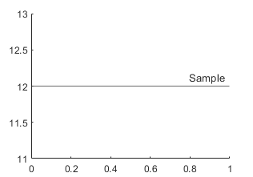`yline(12,'-',{'Sample','x=12'})`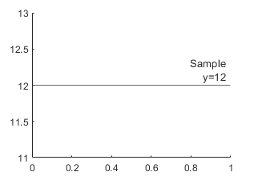#### 为多行创建标签

`yline([13 20 33],'-','Sample')`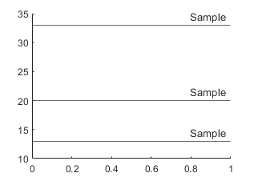```label = {'Sample 1','Sample 2','Sample 3'}; yline([13 20 33],'-',label)```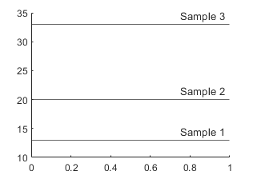```label = {'Sample 1',sprintf('Sample 2\nx=20'),'Sample 3'}; yline([13 20 33],'-',label)```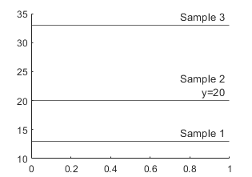### 名称-值对组参数

• RGB 三元组是包含三个元素的行向量，其元素分别指定颜色中红、绿、蓝分量的强度。强度值必须位于 `[0,1]` 范围内，例如 `[0.4 0.6 0.7]`

• 十六进制颜色代码是字符向量或字符串标量，以井号 (`#`) 开头，后跟三个或六个十六进制数字，范围可以是 `0``F`。这些值不区分大小写。因此，颜色代码 `'#FF8800'``'#ff8800'``'#F80'``'#f80'` 是等效的。

`'red'``'r'``[1 0 0]``'#FF0000'``'green'``'g'``[0 1 0]``'#00FF00'``'blue'``'b'``[0 0 1]``'#0000FF'``'cyan'` `'c'``[0 1 1]``'#00FFFF'``'magenta'``'m'``[1 0 1]``'#FF00FF'``'yellow'``'y'``[1 1 0]``'#FFFF00'``'black'``'k'``[0 0 0]``'#000000'``'white'``'w'``[1 1 1]``'#FFFFFF'``'none'`不适用不适用不适用无颜色

RGB 三元组十六进制颜色代码外观
`[0 0.4470 0.7410]``'#0072BD'``[0.8500 0.3250 0.0980]``'#D95319'``[0.9290 0.6940 0.1250]``'#EDB120'``[0.4940 0.1840 0.5560]``'#7E2F8E'``[0.4660 0.6740 0.1880]``'#77AC30'``[0.3010 0.7450 0.9330]``'#4DBEEE'``[0.6350 0.0780 0.1840]``'#A2142F'``'right'`线的右侧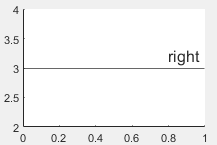`'left'`线的左侧`'center'`线的中心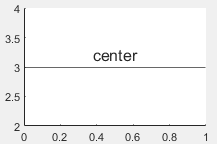`'top'`线的顶部。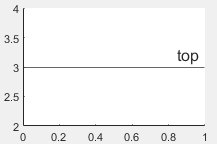`'middle'`线的中间。标签将线分段。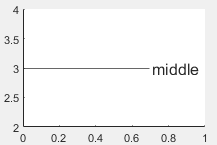`'bottom'`线的底部。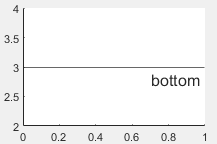`'aligned'`标签与指定线的方向相同。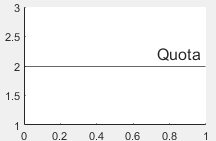`'horizontal'`无论线的方向如何，标签始终为水平方向。